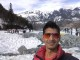# How to transpose an array in PHP with irregular subarray size?

```    function transposeCsvData(\$data)
{
\$ct=0;
foreach(\$data as \$key => \$val)
{
//echo count(\$val);
if(\$ct< count(\$val))
\$ct=count(\$val);
}
//echo \$ct;
\$blank=array_fill(0,\$ct,array_fill(0,count(\$data),null));
//print_r(\$blank);

\$retData = array();
foreach (\$data as \$row => \$columns)
{
foreach (\$columns as \$row2 => \$column2)
{
\$retData[\$row2][\$row] = \$column2;
}
}
\$final=array();
foreach(\$retData as \$k=>\$aval)
{
\$final[]=array_replace(\$blank[\$k], \$aval);
}
return \$final;
}
```

Test Code

```\$a[]=array("name","john","sam","patrick");
\$a[]=array("color","orange","green");
\$a[]=array("bikes","suzuki","audi","mercedes","bmw","volks","rover");
\$a[]=array("techgiants","samsung","amazon");

print_r(transposeCsvData(\$a));
```

Output

```Array
(
 => Array
(
 => name
 => color
 => bikes
 => techgiants
)

 => Array
(
 => john
 => orange
 => suzuki
 => samsung
)

 => Array
(
 => sam
 => green
 => audi
 => amazon
)

 => Array
(
 => patrick
 =>
 => mercedes
 =>
)

 => Array
(
 =>
 =>
 => bmw
 =>
)

 => Array
(
 =>
 =>
 => volks
 =>
)

 => Array
(
 =>
 =>
 => rover
 =>
)

)
```## Author: Harshvardhan Malpani

PHP Developer based in New Delhi, India. Working as a freelance web developer providing server deployment, website development and maintenance services.